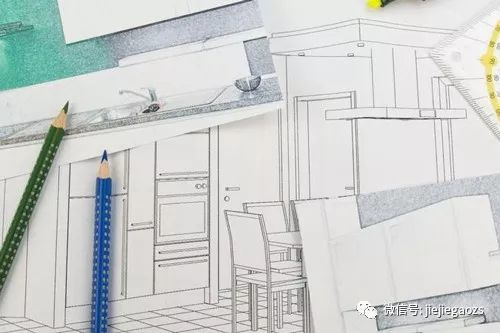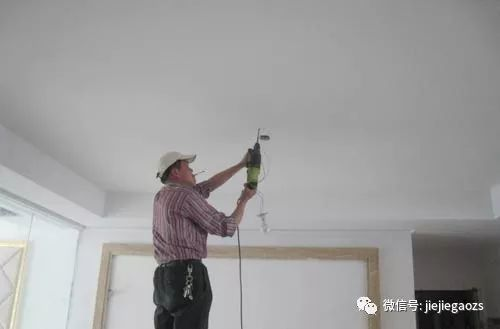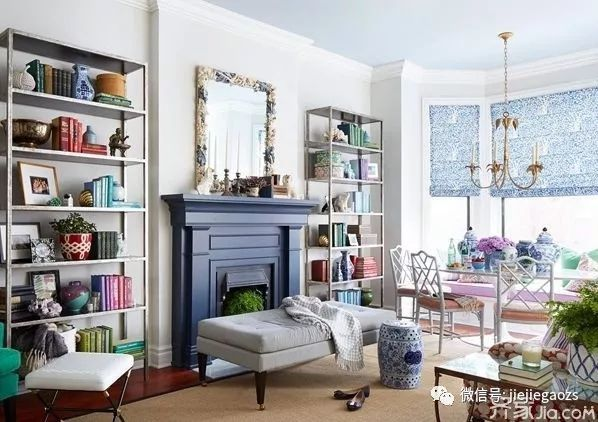|

# 【步骤】自己怎么装修房子 自己装修房子的步骤详解

1前期设计--2主体拆改--3水电改造--4木工--5贴砖--6刷墙面漆--7厨卫吊顶--8橱柜安装--9木门安装--10地板安装--11铺贴壁纸--12散热器安装--13开关插座安装--14灯具安装--15五金洁具安装--16窗帘杆安装--17拓荒保洁--18家具进场--19家电安装--20家居完成，大致是按照这20步完成的。1、明确装修过程涉及的面积。特别是贴砖面积、墙面漆面积、壁纸面积、地板面积；

2、明确主要墙面尺寸。特别是以后需要设计摆放家具的墙面尺寸。1、看看油烟机插座的位置是否影响以后油烟机的安装；

2、看看水表的位置是否合适；

3、看看上水口的位置是否便于以后安装水槽。

1、过门石、大理石窗台的安装。过门石的安装可以和铺地砖一起完成，也可以在铺地砖之后，大理石窗台的安装一般在窗套做好之后，安装大理石的工人会准备玻璃胶，顺手就把大理石和窗套用玻璃胶封住了。

2、地漏的安装。地漏是家装五金件中第一个出场的，因为它要和地砖共同配合安装。所以，朋友们在开始逛建材的时候，应该赶早儿买地漏。

3、油烟机的安装。油烟机是家电第一个出场的，厨房墙地砖铺好之后，就可以考虑安装油烟机了。

“瓦工老二”离场，这时候可以约橱柜第二次测量了，准确地说，在厨房墙地砖贴完并安装完油烟机之后，就可以约橱柜第二次测量。

“油工老三”进场，主要完成墙面基层处理、刷面漆、给“木工老大”打的家具上漆等工作。准备贴壁纸的朋友们，只需要让“油工老三”在计划贴壁纸的墙面做基层处理就可以。1、地板安装之前，最好让厂家上门勘测一下地面是否需要找平或局部找平，有的装修公司或整修队会建议同学地面找平或局部找平，以地板厂家的实际勘测为准；

2、地板安装之前，家里的铺装地板的地面要清扫干净，要保证地面的干燥，所以清扫过程不要用水。

3、地板安装时，有条件的话，地板的切割一定要在走廊。

1、开关插座不能装在瓷砖的花片和腰线上。

2、开关插座底盒在瓷砖开孔时，边框不能比底盒大2mm以上，也不能开成圆孔。为保证以后安装开关、插座，底盒边应尽量与瓷砖相平，这样以后安装时就不需另找比较长的螺丝。

3、装开关插座的位置不能有两块以上的瓷砖被破坏，并且尽量使其安装在瓷砖正中间。

4、装龙头处开孔必须开成圆孔，不能开成方孔，而且也不能开成“U”型，再在“U”型孔中补一块，开孔的大小不能超过管径的2mm以上，并且出水口边也须与瓷砖平齐。

5、插座安装时，明装插座距地面应不低于1.8米。

6、暗装插座距地面不低于0.3米，为防止儿童触电、用手指触摸或金属物插捅电源的孔眼，一定要选用带有保险挡片的安全插座。

7、单相二眼插座的施工接线要求是：当孔眼横排列时为“左零右火”，竖排列时为“上火下零”。

1、在装灯具时，如果装上分控开关，可以省去很多烦恼。因为如果只有一个总开关，几盏灯同开同闭，就不能选择光线的明暗，也会浪费电能，而装上分控开关可以随时根据需要选择开几盏灯。如果房屋进门处有过道，在过道的末端最好也装一个开关，这样进门后就能直接关掉电源，而不需要再走回门口关灯。

2、照明系统应该设置成可调节的，即当感觉灯光暗淡或者是刺眼的时候，可以进行调整，人们也会感觉更舒服、更自在。3、灯光的色温应该与居室的气氛一致，至少是要与居室的其他区域的色温相似。可以在最上层的地方安装灯具，减少工作时候的阴影。

4、不要只在中央安装单独一个照明光源，应该在厨房中安装一个由不同的灯具和光源组成的多层次的照明系统。

5、安装地点也很重要。直线形的白炽灯或者是荧光灯应该安装在朝向橱柜的前面部分。这样，灯发出的部分光会射向后挡板，然后反射到操作台上，再射向整个空间的中心。1、坐便器的安装顺序

2、洗脸盆的安装顺序

3、浴盆的安装顺序

1、窗帘盒安装时主要是要找好位，画尺寸线认真，预埋件安装准确。安装前做到划线正确，安装量尺必须使标高一致，中心线准确。2、窗帘盒安装时应该对尺寸使两端长度相同。3、要防止窗帘轨道的脱落，一般盖板厚度不宜小于15mm，薄于15mm的盖板应该用机螺丝固定窗帘轨。　4、加工时木材干燥不好，入场后存放受潮，安装时应及时刷油漆一遍。`声明：本文由入驻焦点开放平台的作者撰写，除焦点官方账号外，观点仅代表作者本人，不代表焦点立场错误信息举报电话： 400-099-0099，邮箱：jubao@vip.sohu.com，或点此进行意见反馈，或点此进行举报投诉。`A B C D E F G H J K L M N P Q R S T W X Y Z
A - B - C - D - E
• A
• 鞍山
• 安庆
• 安阳
• 安顺
• 安康
• 澳门
• B
• 北京
• 保定
• 包头
• 巴彦淖尔
• 本溪
• 蚌埠
• 亳州
• 滨州
• 北海
• 百色
• 巴中
• 毕节
• 保山
• 宝鸡
• 白银
• 巴州
• C
• 承德
• 沧州
• 长治
• 赤峰
• 朝阳
• 长春
• 常州
• 滁州
• 池州
• 长沙
• 常德
• 郴州
• 潮州
• 崇左
• 重庆
• 成都
• 楚雄
• 昌都
• 慈溪
• 常熟
• D
• 大同
• 大连
• 丹东
• 大庆
• 东营
• 德州
• 东莞
• 德阳
• 达州
• 大理
• 德宏
• 定西
• 儋州
• 东平
• E
• 鄂尔多斯
• 鄂州
• 恩施
F - G - H - I - J
• F
• 抚顺
• 阜新
• 阜阳
• 福州
• 抚州
• 佛山
• 防城港
• G
• 赣州
• 广州
• 桂林
• 贵港
• 广元
• 广安
• 贵阳
• 固原
• H
• 邯郸
• 衡水
• 呼和浩特
• 呼伦贝尔
• 葫芦岛
• 哈尔滨
• 黑河
• 淮安
• 杭州
• 湖州
• 合肥
• 淮南
• 淮北
• 黄山
• 菏泽
• 鹤壁
• 黄石
• 黄冈
• 衡阳
• 怀化
• 惠州
• 河源
• 贺州
• 河池
• 海口
• 红河
• 汉中
• 海东
• 怀来
• I
• J
• 晋中
• 锦州
• 吉林
• 鸡西
• 佳木斯
• 嘉兴
• 金华
• 景德镇
• 九江
• 吉安
• 济南
• 济宁
• 焦作
• 荆门
• 荆州
• 江门
• 揭阳
• 金昌
• 酒泉
• 嘉峪关
K - L - M - N - P
• K
• 开封
• 昆明
• 昆山
• L
• 廊坊
• 临汾
• 辽阳
• 连云港
• 丽水
• 六安
• 龙岩
• 莱芜
• 临沂
• 聊城
• 洛阳
• 漯河
• 娄底
• 柳州
• 来宾
• 泸州
• 乐山
• 六盘水
• 丽江
• 临沧
• 拉萨
• 林芝
• 兰州
• 陇南
• M
• 牡丹江
• 马鞍山
• 茂名
• 梅州
• 绵阳
• 眉山
• N
• 南京
• 南通
• 宁波
• 南平
• 宁德
• 南昌
• 南阳
• 南宁
• 内江
• 南充
• P
• 盘锦
• 莆田
• 平顶山
• 濮阳
• 攀枝花
• 普洱
• 平凉
Q - R - S - T - W
• Q
• 秦皇岛
• 齐齐哈尔
• 衢州
• 泉州
• 青岛
• 清远
• 钦州
• 黔南
• 曲靖
• 庆阳
• R
• 日照
• 日喀则
• S
• 石家庄
• 沈阳
• 双鸭山
• 绥化
• 上海
• 苏州
• 宿迁
• 绍兴
• 宿州
• 三明
• 上饶
• 三门峡
• 商丘
• 十堰
• 随州
• 邵阳
• 韶关
• 深圳
• 汕头
• 汕尾
• 三亚
• 三沙
• 遂宁
• 山南
• 商洛
• 石嘴山
• T
• 天津
• 唐山
• 太原
• 通辽
• 铁岭
• 泰州
• 台州
• 铜陵
• 泰安
• 铜仁
• 铜川
• 天水
• 天门
• W
• 乌海
• 乌兰察布
• 无锡
• 温州
• 芜湖
• 潍坊
• 威海
• 武汉
• 梧州
• 渭南
• 武威
• 吴忠
• 乌鲁木齐
X - Y - Z
• X
• 邢台
• 徐州
• 宣城
• 厦门
• 新乡
• 许昌
• 信阳
• 襄阳
• 孝感
• 咸宁
• 湘潭
• 湘西
• 西双版纳
• 西安
• 咸阳
• 西宁
• 仙桃
• 西昌
• Y
• 运城
• 营口
• 盐城
• 扬州
• 鹰潭
• 宜春
• 烟台
• 宜昌
• 岳阳
• 益阳
• 永州
• 阳江
• 云浮
• 玉林
• 宜宾
• 雅安
• 玉溪
• 延安
• 榆林
• 银川
• Z
• 张家口
• 镇江
• 舟山
• 漳州
• 淄博
• 枣庄
• 郑州
• 周口
• 驻马店
• 株洲
• 张家界
• 珠海
• 湛江
• 肇庆
• 中山
• 自贡
• 资阳
• 遵义
• 昭通
• 张掖
• 中卫

1室1厅1厨1卫1阳台

1
2
3
4
5

0
1
2

1

1

0
1
2
3报名成功，资料已提交审核A B C D E F G H J K L M N P Q R S T W X Y Z
A - B - C - D - E
• A
• 鞍山
• 安庆
• 安阳
• 安顺
• 安康
• 澳门
• B
• 北京
• 保定
• 包头
• 巴彦淖尔
• 本溪
• 蚌埠
• 亳州
• 滨州
• 北海
• 百色
• 巴中
• 毕节
• 保山
• 宝鸡
• 白银
• 巴州
• C
• 承德
• 沧州
• 长治
• 赤峰
• 朝阳
• 长春
• 常州
• 滁州
• 池州
• 长沙
• 常德
• 郴州
• 潮州
• 崇左
• 重庆
• 成都
• 楚雄
• 昌都
• 慈溪
• 常熟
• D
• 大同
• 大连
• 丹东
• 大庆
• 东营
• 德州
• 东莞
• 德阳
• 达州
• 大理
• 德宏
• 定西
• 儋州
• 东平
• E
• 鄂尔多斯
• 鄂州
• 恩施
F - G - H - I - J
• F
• 抚顺
• 阜新
• 阜阳
• 福州
• 抚州
• 佛山
• 防城港
• G
• 赣州
• 广州
• 桂林
• 贵港
• 广元
• 广安
• 贵阳
• 固原
• H
• 邯郸
• 衡水
• 呼和浩特
• 呼伦贝尔
• 葫芦岛
• 哈尔滨
• 黑河
• 淮安
• 杭州
• 湖州
• 合肥
• 淮南
• 淮北
• 黄山
• 菏泽
• 鹤壁
• 黄石
• 黄冈
• 衡阳
• 怀化
• 惠州
• 河源
• 贺州
• 河池
• 海口
• 红河
• 汉中
• 海东
• 怀来
• I
• J
• 晋中
• 锦州
• 吉林
• 鸡西
• 佳木斯
• 嘉兴
• 金华
• 景德镇
• 九江
• 吉安
• 济南
• 济宁
• 焦作
• 荆门
• 荆州
• 江门
• 揭阳
• 金昌
• 酒泉
• 嘉峪关
K - L - M - N - P
• K
• 开封
• 昆明
• 昆山
• L
• 廊坊
• 临汾
• 辽阳
• 连云港
• 丽水
• 六安
• 龙岩
• 莱芜
• 临沂
• 聊城
• 洛阳
• 漯河
• 娄底
• 柳州
• 来宾
• 泸州
• 乐山
• 六盘水
• 丽江
• 临沧
• 拉萨
• 林芝
• 兰州
• 陇南
• M
• 牡丹江
• 马鞍山
• 茂名
• 梅州
• 绵阳
• 眉山
• N
• 南京
• 南通
• 宁波
• 南平
• 宁德
• 南昌
• 南阳
• 南宁
• 内江
• 南充
• P
• 盘锦
• 莆田
• 平顶山
• 濮阳
• 攀枝花
• 普洱
• 平凉
Q - R - S - T - W
• Q
• 秦皇岛
• 齐齐哈尔
• 衢州
• 泉州
• 青岛
• 清远
• 钦州
• 黔南
• 曲靖
• 庆阳
• R
• 日照
• 日喀则
• S
• 石家庄
• 沈阳
• 双鸭山
• 绥化
• 上海
• 苏州
• 宿迁
• 绍兴
• 宿州
• 三明
• 上饶
• 三门峡
• 商丘
• 十堰
• 随州
• 邵阳
• 韶关
• 深圳
• 汕头
• 汕尾
• 三亚
• 三沙
• 遂宁
• 山南
• 商洛
• 石嘴山
• T
• 天津
• 唐山
• 太原
• 通辽
• 铁岭
• 泰州
• 台州
• 铜陵
• 泰安
• 铜仁
• 铜川
• 天水
• 天门
• W
• 乌海
• 乌兰察布
• 无锡
• 温州
• 芜湖
• 潍坊
• 威海
• 武汉
• 梧州
• 渭南
• 武威
• 吴忠
• 乌鲁木齐
X - Y - Z
• X
• 邢台
• 徐州
• 宣城
• 厦门
• 新乡
• 许昌
• 信阳
• 襄阳
• 孝感
• 咸宁
• 湘潭
• 湘西
• 西双版纳
• 西安
• 咸阳
• 西宁
• 仙桃
• 西昌
• Y
• 运城
• 营口
• 盐城
• 扬州
• 鹰潭
• 宜春
• 烟台
• 宜昌
• 岳阳
• 益阳
• 永州
• 阳江
• 云浮
• 玉林
• 宜宾
• 雅安
• 玉溪
• 延安
• 榆林
• 银川
• Z
• 张家口
• 镇江
• 舟山
• 漳州
• 淄博
• 枣庄
• 郑州
• 周口
• 驻马店
• 株洲
• 张家界
• 珠海
• 湛江
• 肇庆
• 中山
• 自贡
• 资阳
• 遵义
• 昭通
• 张掖
• 中卫• 手机• 分享
• 设计
免费设计
• 计算器
装修计算器
• 入驻
合作入驻
• 联系
联系我们
• 置顶
返回顶部